# Pyrror

Currently in rewriting phase.

Package for uncertainty analysis in Python.
It was developed while I was doing my Bachelor in physics.
The functionality is based on the lab courses.

## Warnings

The function exec is used within some functionalities.
This can cause harm if exploited.

## General introduction

The main classes of the package are Data, Table and Formula.
The package holds functionality for:

• Error/Uncertainty propagation in equations
• Create tables and calculate different types of averages including arithmetic, geometric, modus, ...
• Normal and simplified gauss propagation of uncertainties
• Regressions including covariant matrices as well as automated chi2 test and residuum plot
• Propagation of units within equations

The Data-class holds a mean value and an error.
Furthermore, can a Data carry a Unit.
The basic arithmetic methods including *, /, +, - are implemented.
Using these the error is propagated using simplified gauss.
For a normal propagation use a Formula.
For natural and other constants the Const-class can be used.
A Const also carries a Unit but not an uncertainty.

## Documentation

### controls.py

#### instancemethod

Controls if an instance of a class is modified during a method.
Raises an AttributeError if the instance is changed.
USAGE AS DECORATOR:

class Dummy:

@instancemethod
def fuzz(self, a):
return Dummy(...)


#### type_check

Controls for a given sequence of tuples if each variable has a specific type.
Raises an TypeError if the type is incorrect.
type_check(*args) -> True

USAGE:

>>> type_check((3, int), (1.2, float))
True
>>> type_check([("3", int)])
TypeError: Type of '<class 'str'>' is false! Try <class 'int'> instead


#### list_type_check

Controls if each element of a given list as a given type.
list_type_check(lst, data_type) -> bool

• lst: List[object] = list of objects which should have the same type data_type
• data_type: type = specific type

Returns True if all objects in lst have the type data_type otherwise returns False.
USAGE:

>>> list_type_check([2, 3, 1], int)
True
>>> list_type_check([2, "3", 1], int)
False


### unit.py

#### Unit-class

Represents a unit like 'm' for meter.
Unit(numerator="", denominator="")

• numerator = str represents the upper units in a fraction
• denominator = str represents the lower units a fraction

For each numerator and denominator can a sequence of units be defined as specified by the following sign-EBNF:

S := '"' units '"'
units := unit | unit ';' units
unit := string | string '^' integer


METHODS:

• flip():
inverts the unit which means an exchange of numerator and denominator

SPECIAL OPERATIONS:

types + - * / > < == <= >=
Unit Unit Unit bool

USAGE:

>>> a = Unit("m", "s")
>>> a
m/s
>>> b = Unit("J;s", "m")
>>> b
(J*s)/m
>>> c = a * b
>>> c
J


### unit_helper.py

Assistance functionality for working with units.

#### unit_control

Controls if the given argument other carries the same unit as self.
Raises an TypeError if other does not carry any unit.
Raises an ValueError if other does not carry the same unit.

USAGE AS DECORATOR:

class Dummy:

@unit_control
pass


#### has_unit

Returns if the given variable has a unit attribute.
has_unit(variable) -> bool

• variable = object to check

USAGE:

>>> has_unit(3)
False


### data.py

Provides the Data-class and the Const-class.

#### Data-class

Represents a real measurement with an uncertainty.
Data(value: str, error: str, sign: Union[str, Unit] = "", power: int = 0, n: int = 0)

• value = string of the mean value
• error = string of the uncertainty
• sign = either string or Unit. Since only one string is given the usage differs a bit from the one in Unit.
S := '"' units '"' | '"' units '/' units '"'
units := unit | unit ';' units
unit := string | string '^' integer


The string will be split at '/' into numerator and denominator.

• power = additional factor to compensate pre-factors (eg. km -> 10^3 m -> power = 3).
It is advantageous to work with SI-units only and use power instead of kilo, milli, ...
• n = number of significant digits. Has to be greater than 0. If 0 (or not set seperatly), the number of digits
will be determined from the length of error

SPECIAL OPERATIONS:

types + - * / ** > < == <= >=
Data Data Data Data Data bool bool bool bool bool
Const Data Data Data Data bool bool bool bool bool
int Data Data Data Data Data bool bool bool bool bool
float Data Data Data Data Data bool bool bool bool bool

USAGE:

>>> a = Data("123.456", "1.234", power=-2, sign="m/s")
>>> a
(123.456±1.234)*10^-2 m/s
>>> b = Data("4", "1.00000001", sign="s", n=2)
>>> b
(4.0±1.0) s
>>> c = a * b
>>> c
(4.9±1.2) m


#### Const-class

Represents a natural constant (through SI definition some may have no error, eg. the speed of light), or a measurement with neglected uncertainty.
Const(value: Union[int, float], sign: Union[str, Unit])

• value = value of the constant
• sign = either string or Unit. Since only one string is given the usage differs a bit from the one in Unit.
S := '"' units '"' | '"' units '/' units '"'
units := unit | unit ';' units
unit := string | string '^' integer


The string will be split at '/' into numerator and denominator.

SPECIAL OPERATIONS:

types + - * / ** > < == <= >=
Data Data Data Data Data bool bool bool bool bool
Const Const Const Const Const bool bool bool bool bool
int float float Const Const Const bool bool bool bool bool
float float float Const Const Const bool bool bool bool bool

The addition and subtraction with ints, floats is only possible if the Const has a Unit equal to Unit()

USAGE:

>>> a = Const(1, "m")
>>> a
1.0 m
>>> a + 1
ArithmeticError: Addition of values with different units is not possible
>>> b = Const(1, "")
>>> b
1.0
>>> b + 1
2.0
>>> type(b + 1)
<class 'float'>


### data_helper.py

Assistance functionality for working with Data.

#### digits

Returns the number of significant digits from a given error.
digits(error: Union[str, int, float]) -> int

• error = the error the significant digits should be determined for

The result is the number of significant digits.
USAGE:

>>> digits(1.23)
3
>>> digits("0.0012")
2
>>> digits(99.25)
4


#### round_data

Shortens the value and error of a Data to its length Data.n. Furthermore, it calculates which power should be used in the representation.
round_data(data: Data)

• data = a instance of type Data to round values to correct length

Usage:
It is used inside Data automatically. There should not be any need to use this.

### formula.py

#### Formula

Used for more complex functions and connections of uncertain data as well as a better
uncertainty analysis.
Formula(formula_string: str)

• formula_string = str the given formula. This can be any valid sympy expression.

METHODS:

latex(type_dict: Dict[str: type]) -> str

• type_dict = Dictionary specifying which type each variable in the Formula has
Returns a string of Latex-code of the given Formula

show_error(type_dict: Dict[str: type], unicode=False) -> str

• type_dict = Dictionary specifying which type each variable in the Formula has
• unicode = Specifies if unicode should be used
Returns a string of the formula and the propagation formula for the uncertainty

calc_unit(value_dict: Dict[Data, Const, float, int]) -> Unit

• value_dict = Dictionary assign a python object to each variable in the Formula
Returns a Unit for the result. If the Unit could not be determined shows a warning

calc(value_dict: Dict[Data, Const, float, int]) -> Unit

• value_dict = Dictionary assign a python object to each variable in the Formula
Returns the result of the calculation. If the Unit could not be determined shows a warning

USAGE:

>>> f = Formula("x**2 + exp(y)")
>>> f.latex({'x': Data, 'y': Data})
('x^{2} + e^{y}', '\sqrt{4 \left(__delta^{x}\right)^{2} x^{2} + \left(__delta^{y}\right)^{2} e^{2 y}}')
>>> a = Data("2.12", "0.05")
>>> b = Data("1.23", "0.02")
>>> f.calc({'x': a, 'y': b})
(79±2)*10^-1
>>>
>>> f2 = Formula("x**2 + 2 * y")
>>> x = Data("1.3", "0.3", sign="m")
>>> y = Data("0.1", "0.22", sign="m^2")
>>> f2.calc({'x': x, 'y': y})
(19±9)*10^-1 m^2
>>>
>>> f = Formula("acos((2*x-1) / sin(y))")
>>> f
acos((2*x-1) / sin(y))
>>> f.show_error({"x": Data, "y": Data})


Due to problems with markdown is a screenshot of the result given bellow:

### regression.py

Holds classes for different types of regressions.
For implementing new Regressions an abstract base class Regression is given.

#### Regression(ABC)

Abstract base class for regressions. All regressions have to inherit from Regression.
Regression(tab: Table, data_dict: Dict[str, int], n_o_f_p: int)

• tab = Table holding data for regression
• data_dict = Dictionary specifying what to use for the regression.
The dictionary must specify columns for 'x' and 'y': {'x': int, 'y' : int}
• n_o_f_p = number of free parameters. Specifies the number of parameters which will be determined
within the regression

ABSTRACT-METHODS:

__str__() -> str

• Gives a string representation of thr regression parameters.

calc(x) -> Union[float, int, Data, Const]

• calculates the theoretical expected value at x using the regression results

residues()

• creates a plot of the residues and shows it.

#### SimpleRegression(Regression)

Simple regression ignoring uncertainties of x and y.
SimpleRegression(table: Table, data_dict: Dict[str], n=2)

• tab = Table holding data for regression
• data_dict = Dictionary specifying what to use for the regression.
The dictionary must specify columns for 'x' and 'y': {'x': int, 'y' : int}
• n = number of significant digits for the parameters

The information of the regression include the y-intercept and slope.
They can be accessed through __str__().

USAGE:

>>> b = (2 * random.random() - 1) * 100
>>> a = random.random() * 100
>>> b, a
(-35.60688387713966, 39.481128050640734)
>>> f = lambda x: b * x + a
>>> xs = list(range(10))
>>> ys = [f(x) + random.gauss(0, 10) for x in xs]
>>> tab = Table(column_names=["x", "y"])
>>> for x, y in zip(xs, ys):
>>> r = SimpleRegression(tab, {"x": 0, "y": 1})
>>> r
Regression:
y = a + b * x
a: (38.4±4.4)
b: (-345.9±8.3)*10^-1
>>> r.residues() # shown bellow
>>> r.plot() # shown bellow

residues plot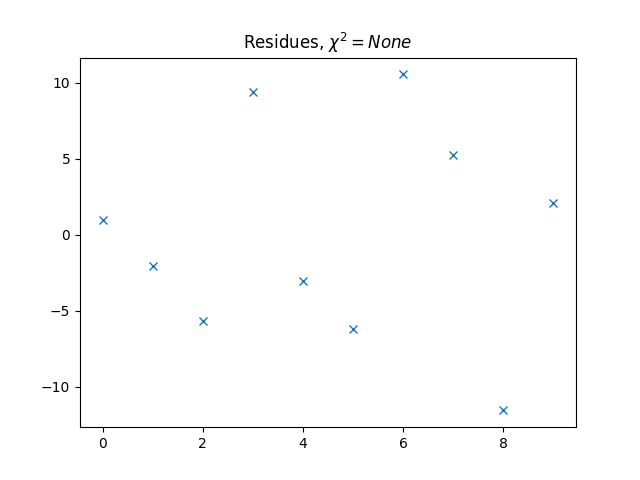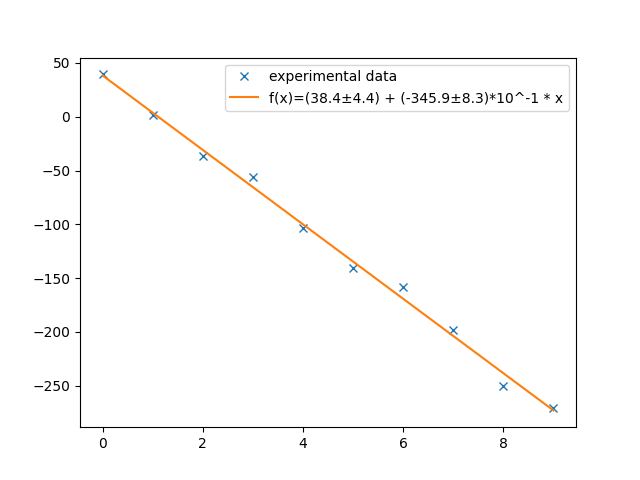#### GaussRegression(Regression)

Simple regression ignoring uncertainties of x and working with uncertainties of y.
GaussRegression(table: Table, data_dict: Dict[str], n=2)

• tab = Table holding data for regression
• data_dict = Dictionary specifying what to use for the regression.
The dictionary must specify columns for 'x' and 'y': {'x': int, 'y' : int}
• n = number of significant digits for the parameters

The information of the regression include the y-intercept, slope, reduced chi2, chi2 and a probability for
such a chi2.
They can be accessed through __str__().

USAGE:

>>> b = (2 * random.random() - 1) * 100
>>> a = random.random() * 100
>>> b, a
(-57.571015317118835, 60.44085386096929)
>>> f = lambda x: b * x + a
>>> xs = [Const(str(x), sign="s") for x in range(15)]
>>> ys = [Data(str(f(x.value) + random.gauss(0, 20)), "20", n=2, sign="m") for x in xs]
>>> tab = Table(column_names=["x", "y"], signs=["s", "m"])
>>> for x, y in zip(xs, ys):
>>> r = GaussRegression(tab, {"x": 0, "y": 1})
>>> r
Regression:
y = a + b * x
a: (67.4±9.8) m
b: (-57.7±1.2) m/s
Chi2     : 10.127125000000001, Probability: 0.684, Chi2 red.: 0.7790096153846154
>>> r.residues() # shown bellow
>>> r.plot() # shown bellow

residues plot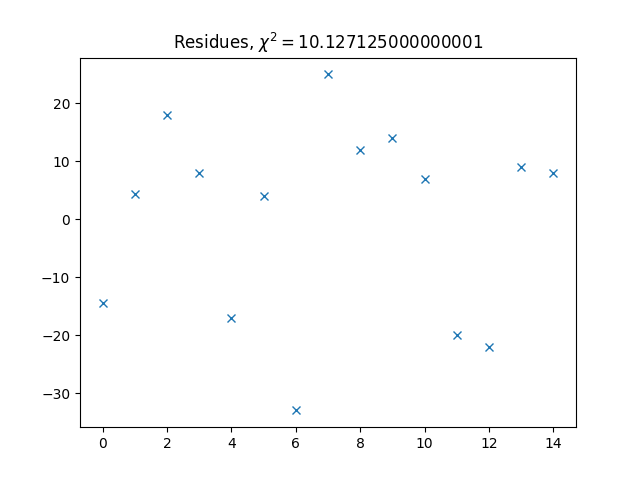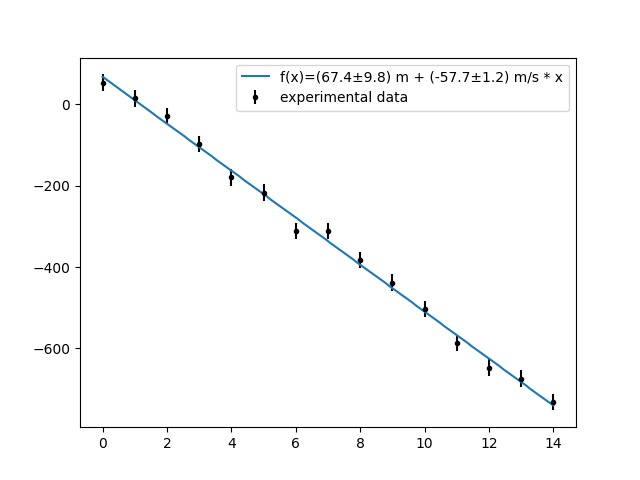#### CovRegression(Regression)

!!!Attention: Unfortunatly, does this class has a bug in calculating the error with the .calc()-Method!!!
Simple regression ignoring uncertainties of x and working with uncertainties of y.
CovRegression(formula_string: str, table: Table, data_dict: Dict[str, int], pars: List[str], n=2)

formula_string = A string specifying a formula which should be fitted.
The format of a formula is specified as given by the formula-EBNF.

S := 'y = ' exprs
exprs := expr | expr '+' exprs | expr '-' exprs
expr := para '*' func | para
para := char ?has to be unique?
func := ?mathematical function of x. Has to be a valid sympy expression?


The ? start and end EBNF-extensions.

table = Table holding data for regression

data_dict = Dictionary specifying what to use for the regression.
The dictionary must specify columns for 'x' and 'y': {'x': int, 'y' : int}

pars = List of the used parameters

n = number of significant digits for the parameters

The information of the regression include the parameters, reduced chi2, chi2 and a probability for
such a chi2.
They can be accessed through __str__().

USAGE:

>>> data = [
-0.849, -0.738, -0.537, -0.354, -0.196,
-0.019, 0.262, 0.413, 0.734, 0.882,
1.258, 1.305, 1.541, 1.768, 1.935,
2.147, 2.456, 2.676, 2.994, 3.200,
3.318]
>>> datas = [(Const(i, sign="°C"), Data(str(data[i // 5]), "0.05", sign="mV")) for i in range(0, 105, 5)]
>>> tab = Table(columns=2, column_names=["x", "y"], signs=["°C", "mV"])
>>> for data in datas:
>>> r = CovRegression("y = a + b*x + c*x**2", tab, {"x": 0, "y": 1}, ["a", "b", "c"])
Regression:
y =  a + b*x + c*x**2
a: -0.919926595143981
b: 0.0376650836577612
c: 0.0000551933192665457
Chi2     : 26.32205952, Probability: 0.093, Chi2 red.: 1.46233664


residues plot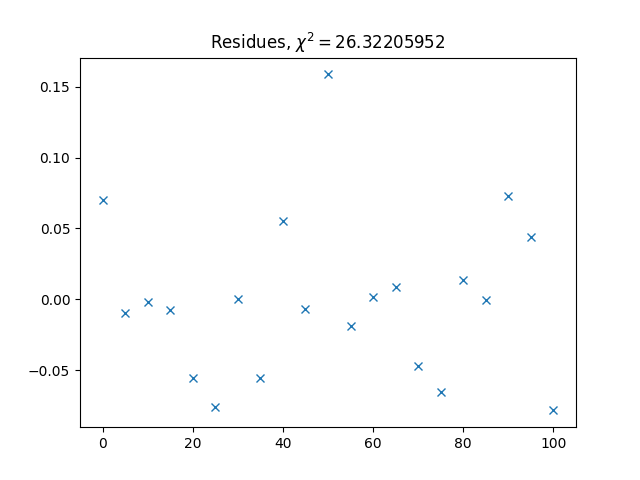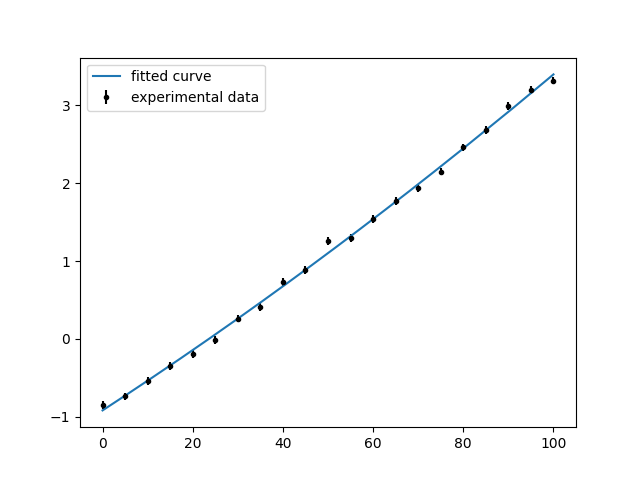### chi_2.py

#### Chi2

Calculates the reduced chi2_red, the chi2, and the probability for a Regression.
Chi2(reg: Regression, chi2=True)

• reg = Regression to calculate chi2 and residues for
• chi2 = specifies if chi2 should be calculated or just the residues

METHODS:

• show_residues()
• creates and shows a plot of the residues

USAGE:

This class is automatically used within a Regression.
It should not be necessary to use it manually.

### table.py

#### Table

Class for storing many relevant data of different types. It can perform calculations columnwise and simplifies the
handling of the calculations.
Table(column_names: List[str] = [], columns=2, signs: List[Union[str, Unit]] = [])

• column_names = List of strings representing the names for the column
• columns = Number of columns for the table
• signs = List of units defined as given by the sign-EBNF:
    S := '"' units '"' | '"' units '/' units '"'
units := unit | unit ';' units
unit := string | string '^' integer


METHODS:

calc(formula: Formula, para_dict: Dict[str: Union[int, str, Any]], column_name="", sign=True)
Uses the given formula on each row of the table to calculate a new column.

• formula = Formula to use for calculation
• para_dict = Dictionary specifying how the parameters are set.
How the parameter will be treated depends on the type of the value.
• int: The value will be used as column index, and the parameter will be set row-wise
• str: The value will be used as value using float(value)
• Any: All other types will be used as they are

add(data_tuple: Tuple[Any])
Adds a new data-row to the Table. All columns have to be set. Use an empty string if you wish to fill it later.

• data_tuple = Tuple of data to add in.
If a Data or Const is inserted it must have the same Unit as the column or Unit()

delete(line_index: int)
Deletes a data-row in the Table.

• line_index = The line to delete.

drop(line_index: int) -> List[Any]
Deletes a data-row in the Table and returns it.

• line_index = The line to delete.

add_column(name="")
Adds a new column to the Table.

• name = Name of the new column. If not set the name will be 'Column <i>' with i is the number of columns.

insert(line: int, column: int, value: Any)
Inserts a value at line, column.

• line = The line the value has to be inserted
• column = The column the value has to be inserted
• value = The value to insert into the Table. If the value contains a Unit it will be replaced by the
one corresponding to the column.

export(path: str, name: str, replace_dot=False)
Exports the Table in '.csv'-format.

• path = path were the table should be saved
• name = name of the file. Should end with '.csv'
• replace_dot = Specifies if a '.' or a ',' is used to separate the decimals

max() -> List[Any]
Returns the max of each column.
The type of each element in the list depend on the types in the column.

min() -> List[Any]
Returns the min of each column.
The type of each element in the list depend on the types in the column.

arithmetic_average() -> List[Data]
Returns the arithmetic average of all columns (mean + std).
The type of each element in the list depend on the types in the column.
If the type of the column is Data the average will be a weighted mean with its error.
Otherwise the uncertainty will be the sample standard deviation.

geometric_average() -> List[Union[Unit, float]]
Returns the geometric average of all columns (mean only).
The type of each element depend on the Unit of the column an will either be a float or Const.

harmonic_average() -> List[Union[Unit, float]]
Returns the harmonic average of all columns (mean only).
The type of each element depend on the Unit of the column an will either be a float or Const.

median() -> List[Union[Unit, float]]
Returns the median of all columns (mean only).
The type of each element depend on the Unit of the column an will either be a float or Const.

modus() -> List[Union[Unit, float]]
Returns the modus of all columns (mean only).
The type of each element depend on the Unit of the column an will either be a float or Const.

USAGE:

>>> data = tuple(zip([1, 1, 3, 2], [random.random() for i in range(4)]))
>>> tab = Table(column_names=["x", "y"], columns=2)
>>> for element in data:
>>> tab
x    |y                      |
------------------------------
1    |0.9650392731516132     |
1    |0.42768822918919414    |
3    |0.0979070782420336     |
2    |0.12872070585370476    |
True
>>> tab
x    |y                      |Column 2 [m/s]    |
-------------------------------------------------
1    |0.9650392731516132     |                  |
1    |0.42768822918919414    |                  |
3    |0.0979070782420336     |                  |
2    |0.12872070585370476    |                  |
>>> data = [Data(str(random.randint(1, 20)), str(random.random()), n=2) for i in range(4)]
>>> for index, data_ in enumerate(data):
tab.insert(index, 2, data_)
>>> tab
x    |y                      |Column 2 [m/s]        |
-----------------------------------------------------
1    |0.9650392731516132     |(170.0±9.4)*10^-2     |
1    |0.42768822918919414    |(1300.0±3.8)*10^-4    |
3    |0.0979070782420336     |(60.0±6.5)*10^-2      |
2    |0.12872070585370476    |(40.0±3.1)*10^-2      |
>>> f = Formula("a * c")
>>> tab.calc(f, {"a": 1, "c": Const(1, "s")}, "time")
>>> tab
x    |y                      |Column 2 [m/s]        |time [s]               |
-----------------------------------------------------------------------------
1    |0.9650392731516132     |(170.0±9.4)*10^-2     |0.9650392731516132     |
1    |0.42768822918919414    |(1300.0±3.8)*10^-4    |0.42768822918919414    |
3    |0.0979070782420336     |(60.0±6.5)*10^-2      |0.0979070782420336     |
2    |0.12872070585370476    |(40.0±3.1)*10^-2      |0.12872070585370476    |
>>> tab.calc(f, {"a": 2, "c": 3}, "distance")
>>> tab
x    |y                      |Column 2 [m/s]        |time [s]               |distance [m]         |
---------------------------------------------------------------------------------------------------
1    |0.9650392731516132     |(170.0±9.4)*10^-2     |0.9650392731516132     |(164.1±9.1)*10^-2    |
1    |0.42768822918919414    |(1300.0±3.8)*10^-4    |0.42768822918919414    |(556.0±1.6)*10^-4    |
3    |0.0979070782420336     |(60.0±6.5)*10^-2      |0.0979070782420336     |(58.7±6.4)*10^-3     |
2    |0.12872070585370476    |(40.0±3.1)*10^-2      |0.12872070585370476    |(51.5±4.0)*10^-3     |
>>> tab.arithmetic_average()
[(17.5±4.8)*10^-1, (4.0±2.0)*10^-1, (1300.8±3.8)*10^-4 m/s, (4.0±2.0)*10^-1 s, (556.0±1.6)*10^-4 m]
>>> tab.export(<path>, "dummy.csv") # <path> needs to be a real path


The last order produces a file called 'dummy.csv'. This file is given bellow:

## TODO:

make to python package

Tests for chi_2

Tests for regression

Improve all Tests (they no longer test all functionalities)

Data should format in such a way, that the mean value is given by 1,...
not the error

Refractor Table (access via column_name, access via tab[index], smarter design)

Allow fast access tables for weighted average between data or similar functionality